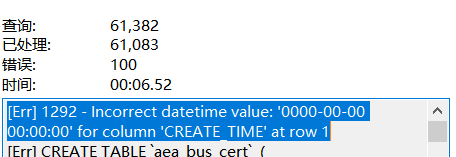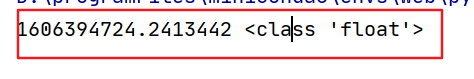Python常用操作的复杂度Stella981
• 阅读 441

我们前面讲过list、deque、堆、字典树等高性能计算的技巧，这一节我们来整理一下Python中常用操作的时间复杂度。本文中的N表示容器的元素数量，K表示参数中元素的数量或参数的值。

• list

lst = list(range(10,20))l1 = list(range(100,105))

lst

O(1)

lst.pop()

O(1)

lst.append(l1)

O(1)

lst.extend(l1)

O(K)

lst.clear()

O(1)

lst.copy()

O(N)

lst.count(15)

O(N)

lst.remove(15)

O(N)

lst.reverse()

O(N)

lst.sort()

O(N*log(N))

lst.insert(1,200)

O(N)

del lst

O(N)

lst.index(15)

O(N)

bisect.bisect_left(lst, 15)

O(log(N))

• tuple

tuple因为不可写，因此操作相对较少

``````tpl = tuple(range(10))
``````

tpl

O(1)

tpl.count(2)

O(N)

tpl.index(2)

O(N)

• set

ss1 = set(range(10))ss2 = set(range(5,15))

5 in ss1

O(N)

ss1 | ss2

O(len(ss1)+len(ss2))

ss1 & ss2

O(len(s)*len(t))

ss1 - ss2

O(len(ss1))

ss1 ^ ss2

O(len(ss1)*len(ss2))

O(1)

ss1.pop()

O(1)

ss1.remove(5)

O(1)

• dict

dd = {'a':10,'b':20,'c':30,'d':40}

dd['e'] = 50

O(1)

dd['a']

O(1)

del dd['a']

O(1)

dd['b'] = 100

O(1)

dd.pop('b')

O(1)

dd.clear()

O(1)

• deque

``````from collections import dequedeq = deque(range(10))ll = list(range(10))
``````

deq.pop()

O(1)

deq.popleft()

O(1)

deq.append(1)

O(1)

deq.appendleft(1)

O(1)

deq.extend(ll)

O(K)

deq.extendleft(ll)

O(K)

deq.rotate(K)

O(K)

deq.remove(5)

O(N)

deq

O(1)

deq[N-1]

O(1)

deq[N/2]

O(N)

Python高性能系列文章blmius
1年前
MySQL:[Err] 1292 - Incorrect datetime value: ‘0000-00-00 00:00:00‘ for column ‘CREATE_TIME‘ at row 1Karen110
1年前
​一篇文章总结一下Python库中关于时间的常见操作Stella981
11个月前
List的Select 和Select().tolist()
List<Person> delp = new List<Person> { new Person{ Id=1,Name="小明1",Age=11,Sign=0 }, new Person{ Id=2,Name="小明2",Age=12 ,Stella981
11个月前
Python之time模块的时间戳、时间字符串格式化与转换
Python处理时间和时间戳的内置模块就有`time`，和`datetime`两个，本文先说`time`模块。 ### 关于时间戳的几个概念 * 时间戳，根据1970年1月1日00:00:00开始按秒计算的偏移量。 * 时间元组（`struct_time`），包含9个元素。  `time.struct_time(tm_yEaster79
11个月前Wesley13
11个月前
java常用类（2）Stella981
11个月前
### **设置在列表中显示的字段，id为django模型默认的主键** list_display = ('id', 'name', 'sex', 'profession', 'email', 'qq', 'phone', 'status', 'create_time') ### **设置在列表可编辑字段** list_editableWesley13
11个月前
PHP中的NOW（）函数Wesley13
11个月前
MySQL部分从库上面因为大量的临时表tmp_table造成慢查询
#### 背景描述 # Time: 2019-01-24T00:08:14.705724+08:00 # User@Host: **[**] @ [**] Id: ** # Schema: sentrymeta Last_errno: 0 Killed: 0 # Query_time: 0.315758 Lock_helloworld_34035044
2个月前

​在我们用皕杰报表工具设计填报报表时，如何在新增行里自动增加id呢？能新增整数排序id吗？目前可以在新增行里自动增加id，但只能用uuid函数增加UUID编码，不能新增整数排序id。 uuid函数说明：获取一个UUID，可以在填报表中用来创建数据ID语法：uuid() 或 uuid(sep)参数说明：sep 布尔值，生成的uuid中是否包含分隔符''，缺省为Stella981
Lv1

1.7w

16

4